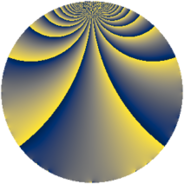# Properties

 Label 1045.2.iLevel $1045$ Weight $2$ Character orbit 1045.i Rep. character $\chi_{1045}(771,\cdot)$ Character field $\Q(\zeta_{3})$ Dimension $128$ Sturm bound $240$

# Related objects

## Defining parameters

 Level: $$N$$ $$=$$ $$1045 = 5 \cdot 11 \cdot 19$$ Weight: $$k$$ $$=$$ $$2$$ Character orbit: $$[\chi]$$ $$=$$ 1045.i (of order $$3$$ and degree $$2$$) Character conductor: $$\operatorname{cond}(\chi)$$ $$=$$ $$19$$ Character field: $$\Q(\zeta_{3})$$ Sturm bound: $$240$$

## Dimensions

The following table gives the dimensions of various subspaces of $$M_{2}(1045, [\chi])$$.

Total New Old
Modular forms 248 128 120
Cusp forms 232 128 104
Eisenstein series 16 0 16

## Trace form

 $$128 q + 8 q^{3} - 60 q^{4} - 4 q^{6} + 24 q^{7} - 56 q^{9} + O(q^{10})$$ $$128 q + 8 q^{3} - 60 q^{4} - 4 q^{6} + 24 q^{7} - 56 q^{9} - 4 q^{10} - 48 q^{12} - 20 q^{13} + 8 q^{15} - 52 q^{16} - 4 q^{17} - 12 q^{19} - 16 q^{21} + 4 q^{22} + 4 q^{23} - 64 q^{25} + 48 q^{26} - 64 q^{27} + 8 q^{29} + 48 q^{31} - 20 q^{32} + 8 q^{33} - 16 q^{34} - 60 q^{36} + 16 q^{37} - 20 q^{38} - 48 q^{39} + 24 q^{40} + 8 q^{41} - 4 q^{42} - 32 q^{43} + 16 q^{45} - 24 q^{46} + 16 q^{47} + 96 q^{48} + 184 q^{49} + 16 q^{51} - 56 q^{52} + 24 q^{53} - 16 q^{54} + 136 q^{56} + 80 q^{57} + 80 q^{58} - 12 q^{59} + 12 q^{60} - 12 q^{62} - 36 q^{63} - 48 q^{64} - 20 q^{66} - 16 q^{67} - 88 q^{68} + 16 q^{69} + 8 q^{70} - 20 q^{71} - 56 q^{72} - 48 q^{73} - 32 q^{74} - 16 q^{75} + 88 q^{76} + 84 q^{78} - 28 q^{79} - 16 q^{80} - 40 q^{81} + 4 q^{82} - 96 q^{83} + 264 q^{84} - 16 q^{85} - 52 q^{86} - 48 q^{87} - 24 q^{88} - 24 q^{89} - 52 q^{90} + 16 q^{91} + 16 q^{92} + 24 q^{93} + 56 q^{94} + 24 q^{95} - 192 q^{96} + 68 q^{97} + 72 q^{98} + O(q^{100})$$

## Decomposition of $$S_{2}^{\mathrm{new}}(1045, [\chi])$$ into newform subspaces

The newforms in this space have not yet been added to the LMFDB.

## Decomposition of $$S_{2}^{\mathrm{old}}(1045, [\chi])$$ into lower level spaces

$$S_{2}^{\mathrm{old}}(1045, [\chi]) \cong$$ $$S_{2}^{\mathrm{new}}(95, [\chi])$$$$^{\oplus 2}$$$$\oplus$$$$S_{2}^{\mathrm{new}}(209, [\chi])$$$$^{\oplus 2}$$